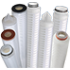• Get FREE shipping on orders over \$99

# Pleated Membrane Filter Cartridges

#### Ordering Guide

Media Absolute Micron Rating End Caps Seal LengthPleated Membrane Cartridges SP-PTFE = PTFE Pleated Membrane
SP-PES = PES Pleated Membrane
SP-N6 = Nylon Pleated Membrane
010 = 0.1 μm
020 = 0.2 μm
045 = 0.45 μm
065 = 0.65 μm
• O = Double Open End
• 7 = Fin (Spear) + 226 O-rings
• 8 = Fin (Spear) + 222 O-rings
• 3 = Flat Closed + 222 O-rings
• 2 = Flat Closed + 226 O-rings
• F = Flat Closed + 215 O-rings
• S = Flat Closed + O-ring Insert
E = EPDM
N = Buna
S = Silicone
V = Viton
10 = 10 inch
20 = 20 inch
30 = 30 inch
40 = 40 inch
SP-PPL = Polypropylene Pleated Membrane 010 = 0.1 μm
020 = 0.2 μm
045 = 0.45 μm
100 = 1 μm
300 = 3 μm
H10 = 10 μm
H100 = 100 μm
*Custom micron ratings between 0.1 and 105 micron can be requested using the same format
5 = 5 inch
10 = 10 inch
20 = 20 inch
30 = 30 inch
40 = 40 inch
Example: SP-PTFE0657N30

#### End Cap Style Guide

O
DOE (Double Open End)
7
Fin (Spear) + 226 O-rings
8
Fin (Spear) + 222 O-rings
3
Flat Closed + 222 O-rings
2
Flat Closed + 226 O-rings
F
Flat Closed + 215 O-rings
S
Flat Closed + O-ring Insert